# READING Unit 8 Unit 19 Unit 20 Unit

• Slides: 29READING Unit 8, Unit 19, Unit 20, Unit 21, Unit 22, Unit 23 �Potential Energy • You can think of potential energy as stored energy, energy ready to be converted into another form • Gravitational potential energy is the energy stored as a result of an object being lifted upwards against the pull of gravity • Potential energy is released when the object is put into motion, or allowed to fall.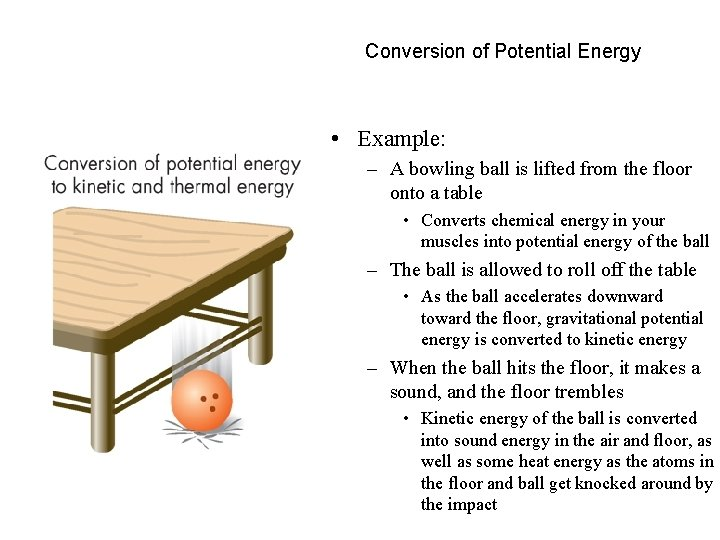Conversion of Potential Energy • Example: – A bowling ball is lifted from the floor onto a table • Converts chemical energy in your muscles into potential energy of the ball – The ball is allowed to roll off the table • As the ball accelerates downward toward the floor, gravitational potential energy is converted to kinetic energy – When the ball hits the floor, it makes a sound, and the floor trembles • Kinetic energy of the ball is converted into sound energy in the air and floor, as well as some heat energy as the atoms in the floor and ball get knocked around by the impactDefinition of Angular Momentum • Angular momentum is the rotational equivalent of inertia • Can be expressed mathematically as the product of the objects mass, rotational velocity, and radius • If no external forces are acting on an object, then its angular momentum is conserved, or a constant: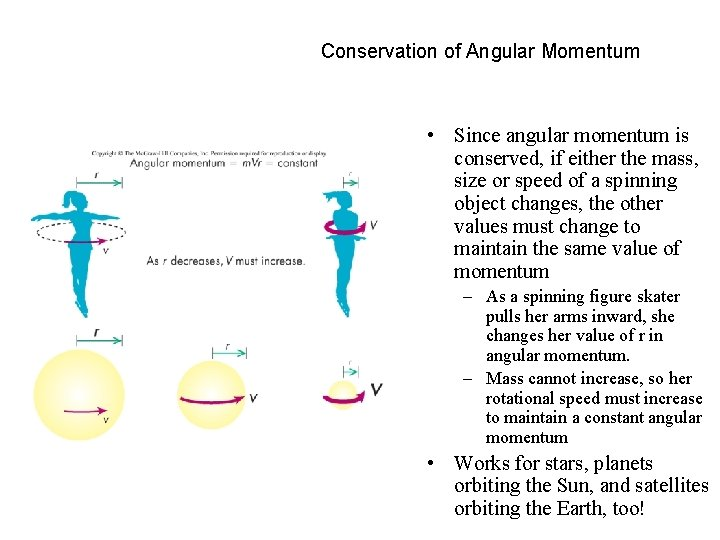Conservation of Angular Momentum • Since angular momentum is conserved, if either the mass, size or speed of a spinning object changes, the other values must change to maintain the same value of momentum – As a spinning figure skater pulls her arms inward, she changes her value of r in angular momentum. – Mass cannot increase, so her rotational speed must increase to maintain a constant angular momentum • Works for stars, planets orbiting the Sun, and satellites orbiting the Earth, too!The Origin of Tides • The Moon exerts a gravitational force on the Earth, stretching it! – Water responds to this pull by flowing towards the source of the force, creating tidal bulges both beneath the Moon and on the opposite side of the EarthSolar Eclipses • At New Moon, the Moon is between the Earth and the Sun. Sometimes, the alignment is just right, allowing the Moon to block the light from the Sun, creating an eclipseSolar Eclipse – the Shadow of the Moon A solar eclipse seen from space • • • In a solar eclipse, the Moon casts a shadow on the surface of the Earth. People within the shadow see the eclipse, and those outside the shadow do not. The Moon’s umbra is the darkest part of the shadow, directly behind the body of the Moon. Within the umbra, the Sun appears completely eclipsed (total eclipse). The penumbra of the Moon (not shown in figure) is the part of the shadow where the light from the Sun is only partially blocked (partial eclipse).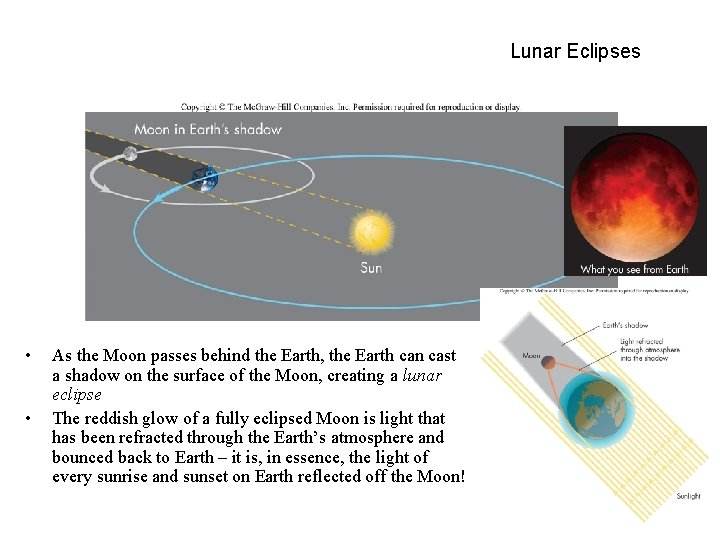Lunar Eclipses • • As the Moon passes behind the Earth, the Earth can cast a shadow on the surface of the Moon, creating a lunar eclipse The reddish glow of a fully eclipsed Moon is light that has been refracted through the Earth’s atmosphere and bounced back to Earth – it is, in essence, the light of every sunrise and sunset on Earth reflected off the Moon!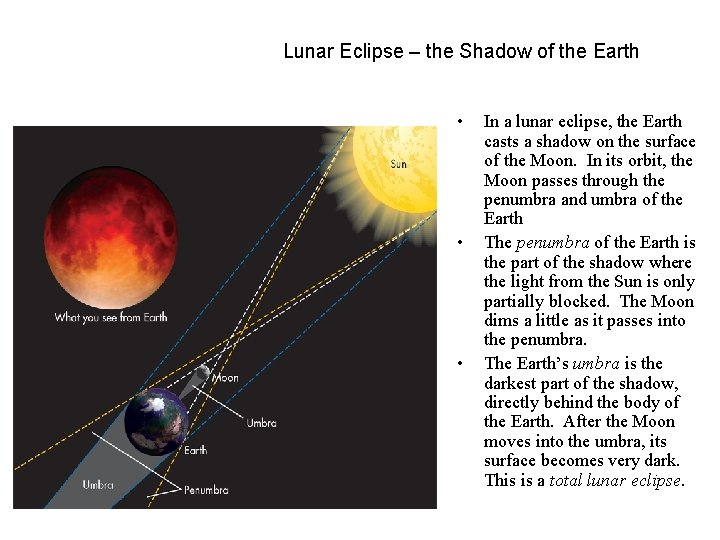Lunar Eclipse – the Shadow of the Earth • • • In a lunar eclipse, the Earth casts a shadow on the surface of the Moon. In its orbit, the Moon passes through the penumbra and umbra of the Earth The penumbra of the Earth is the part of the shadow where the light from the Sun is only partially blocked. The Moon dims a little as it passes into the penumbra. The Earth’s umbra is the darkest part of the shadow, directly behind the body of the Earth. After the Moon moves into the umbra, its surface becomes very dark. This is a total lunar eclipse.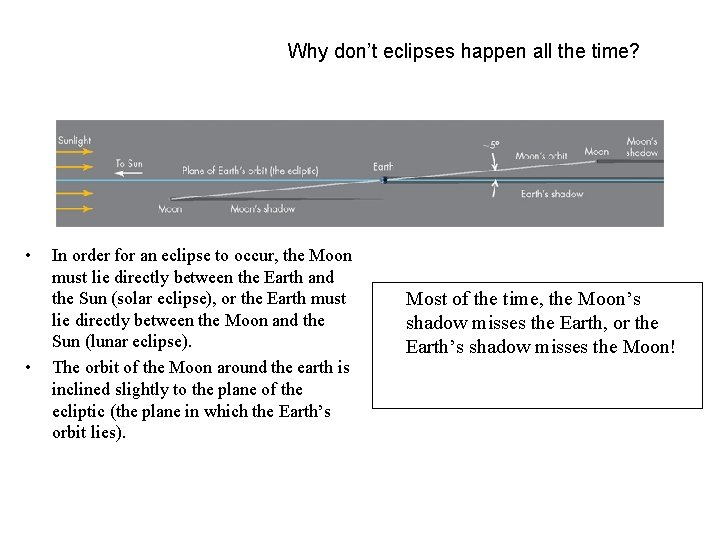Why don’t eclipses happen all the time? • • In order for an eclipse to occur, the Moon must lie directly between the Earth and the Sun (solar eclipse), or the Earth must lie directly between the Moon and the Sun (lunar eclipse). The orbit of the Moon around the earth is inclined slightly to the plane of the ecliptic (the plane in which the Earth’s orbit lies). Most of the time, the Moon’s shadow misses the Earth, or the Earth’s shadow misses the Moon!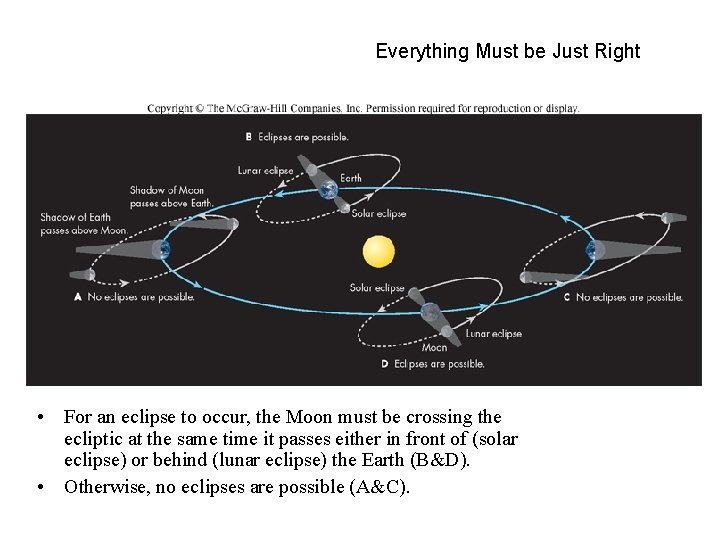Everything Must be Just Right • For an eclipse to occur, the Moon must be crossing the ecliptic at the same time it passes either in front of (solar eclipse) or behind (lunar eclipse) the Earth (B&D). • Otherwise, no eclipses are possible (A&C).The Nature of Light • Light is radiant energy. • Travels very fast – 300, 000 km/sec! • Can be described either as a wave or as a particle traveling through space. • • As a wave… – A small disturbance in an electric field creates a small magnetic field, which in turn creates a small electric field, and so on… • Light propagates itself “by its bootstraps!” – Light waves can interfere with other light waves, canceling or amplifying them! – The color of light is determined by its wavelength. As a particle… – Particles of light (photons) travel through space. – These photons have very specific energies. that is, light is quantized. – Photons strike your eye (or other sensors) like a very small bullet, and are detected.The Effect of Distance on Light • Light from distant objects seems very dim – Why? Is it because the photons are losing energy? – No – the light is simply spreading out as it travels from its source to its destination – The farther from the source you are, the dimmer the light seems – We say that the object’s brightness, or amount of light received from a source, is decreasing This is an inverse-square law – the brightness decreases as the square of the distance (d) from the source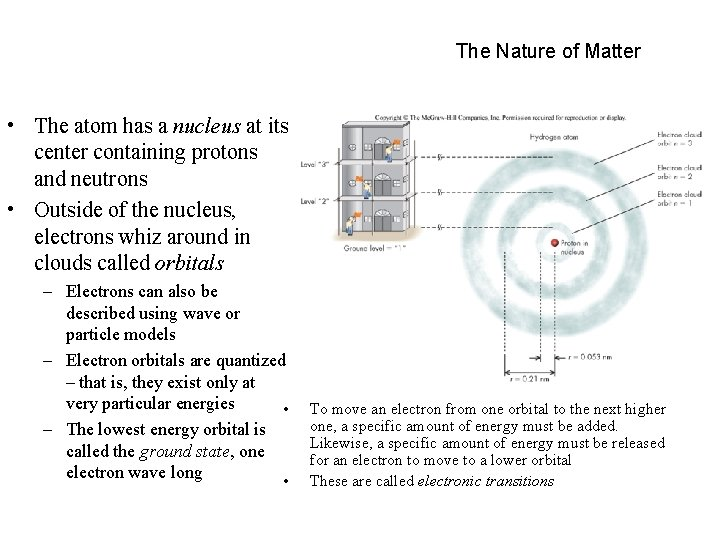The Nature of Matter • The atom has a nucleus at its center containing protons and neutrons • Outside of the nucleus, electrons whiz around in clouds called orbitals – Electrons can also be described using wave or particle models – Electron orbitals are quantized – that is, they exist only at very particular energies • – The lowest energy orbital is called the ground state, one electron wave long • To move an electron from one orbital to the next higher one, a specific amount of energy must be added. Likewise, a specific amount of energy must be released for an electron to move to a lower orbital These are called electronic transitions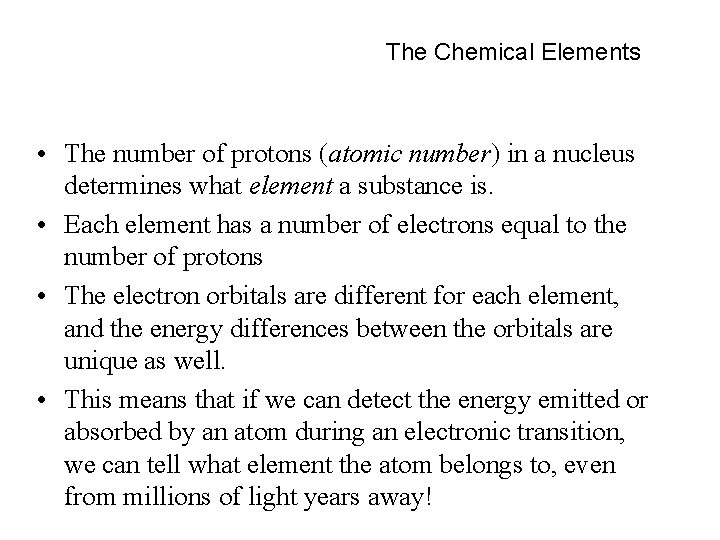The Chemical Elements • The number of protons (atomic number) in a nucleus determines what element a substance is. • Each element has a number of electrons equal to the number of protons • The electron orbitals are different for each element, and the energy differences between the orbitals are unique as well. • This means that if we can detect the energy emitted or absorbed by an atom during an electronic transition, we can tell what element the atom belongs to, even from millions of light years away!Periodic Table D. MendeleevAbsorption • If a photon of exactly the right energy (corresponding to the energy difference between orbitals) strikes an electron, that electron will absorb the photon and move into the next higher orbital – The atom is now in an excited state • If the photon is of higher or lower energies, it will not be absorbed – it will pass through as if the atom were not there. • • This process is called absorption If the electron gains enough energy to leave the atom entirely, we say the atom is now ionized, or is an ion.Emission • If an atom drops from one orbital to the next lower one, it must first emit a photon with the same amount of energy as the orbital energy difference. • This is called emission.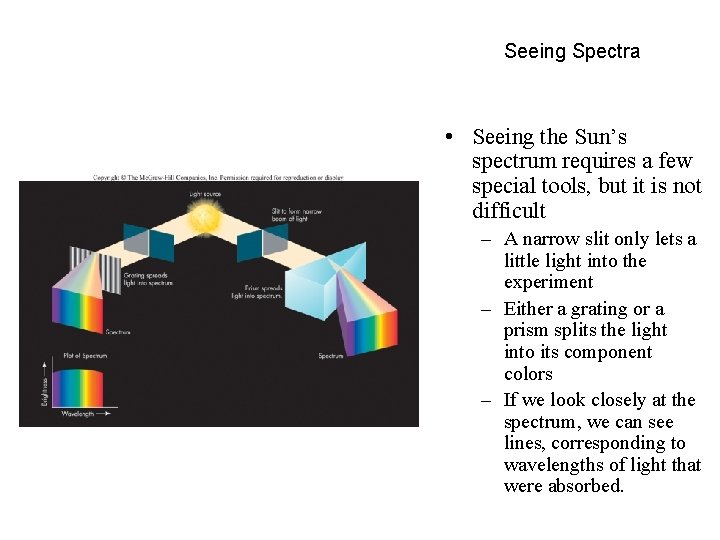Seeing Spectra • Seeing the Sun’s spectrum requires a few special tools, but it is not difficult – A narrow slit only lets a little light into the experiment – Either a grating or a prism splits the light into its component colors – If we look closely at the spectrum, we can see lines, corresponding to wavelengths of light that were absorbed.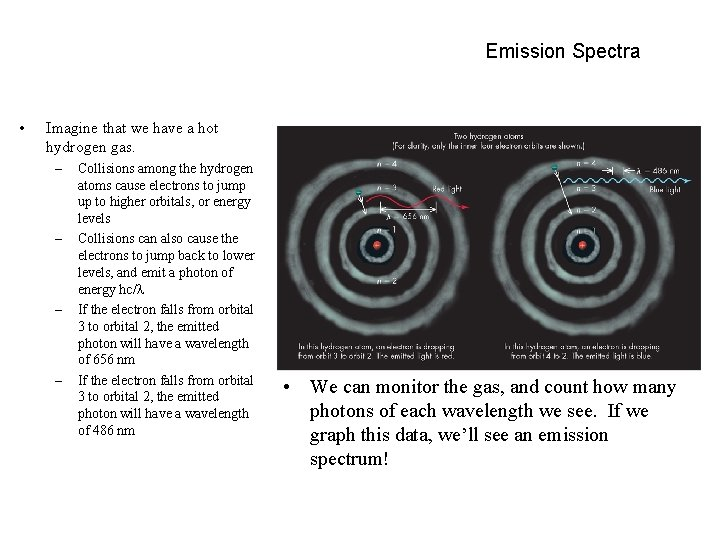Emission Spectra • Imagine that we have a hot hydrogen gas. – – Collisions among the hydrogen atoms cause electrons to jump up to higher orbitals, or energy levels Collisions can also cause the electrons to jump back to lower levels, and emit a photon of energy hc/ If the electron falls from orbital 3 to orbital 2, the emitted photon will have a wavelength of 656 nm If the electron falls from orbital 3 to orbital 2, the emitted photon will have a wavelength of 486 nm • We can monitor the gas, and count how many photons of each wavelength we see. If we graph this data, we’ll see an emission spectrum!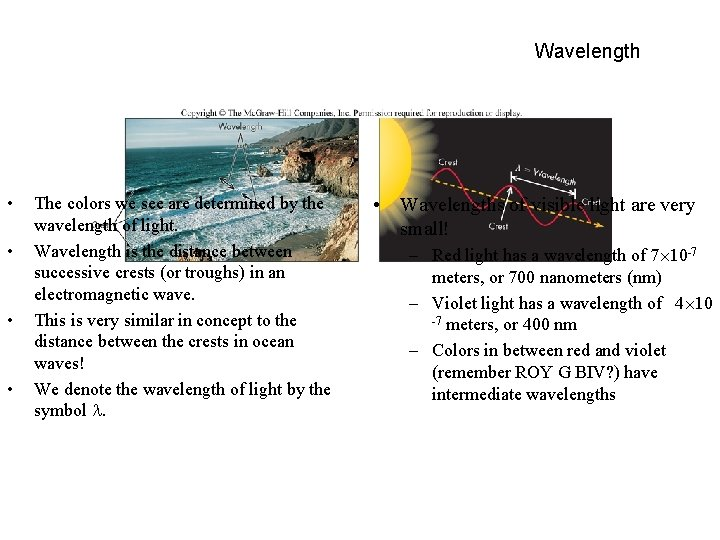Wavelength • • The colors we see are determined by the wavelength of light. Wavelength is the distance between successive crests (or troughs) in an electromagnetic wave. This is very similar in concept to the distance between the crests in ocean waves! We denote the wavelength of light by the symbol . • Wavelengths of visible light are very small! – Red light has a wavelength of 7 10 -7 meters, or 700 nanometers (nm) – Violet light has a wavelength of 4 10 -7 meters, or 400 nm – Colors in between red and violet (remember ROY G BIV? ) have intermediate wavelengthsFrequency • • Sometimes it is more convenient to talk about light in terms of frequency, or how fast successive crests pass by a given point You can think of frequency as a measure of how fast you bob up and down as the waves pass. Frequency has units of Hz (Hertz), and is denoted by the symbol Long wavelength light has a low frequency, and short wavelength light has a high frequency • Frequency and wavelength are related by: ‘c’ is the speed of light.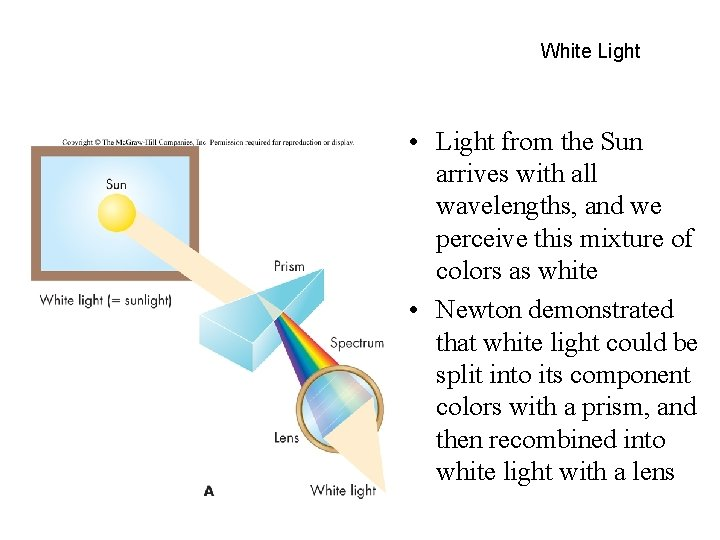White Light • Light from the Sun arrives with all wavelengths, and we perceive this mixture of colors as white • Newton demonstrated that white light could be split into its component colors with a prism, and then recombined into white light with a lensMeasuring Temperature • It is useful to think of temperature in a slightly different way than we are accustomed to – Temperature is a measure of the motion of atoms in an object – Objects with low temperatures have atoms that are not moving much – Objects with high temperatures have atoms that are moving around very rapidly • The Kelvin temperature scale was designed to reflect this – 0 K is absolute zero –the atoms in an object are not moving at all!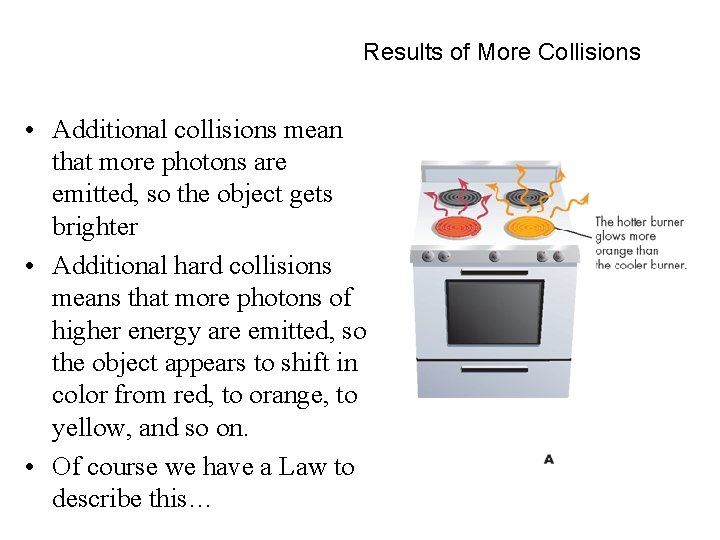Results of More Collisions • Additional collisions mean that more photons are emitted, so the object gets brighter • Additional hard collisions means that more photons of higher energy are emitted, so the object appears to shift in color from red, to orange, to yellow, and so on. • Of course we have a Law to describe this…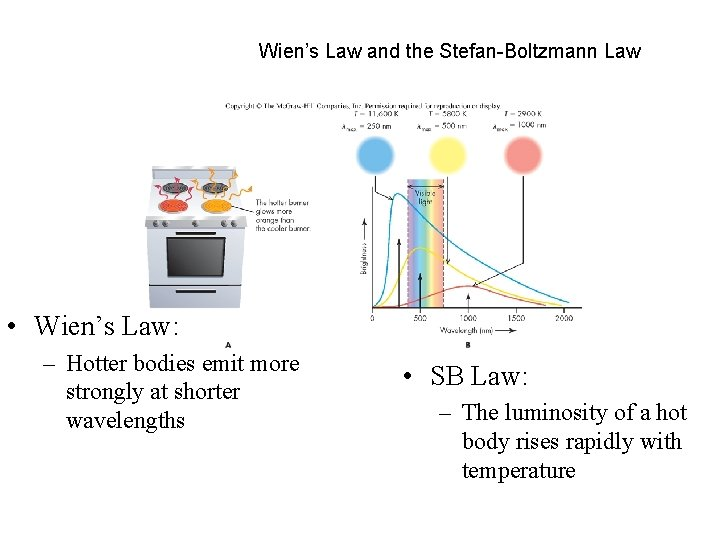Wien’s Law and the Stefan-Boltzmann Law • Wien’s Law: – Hotter bodies emit more strongly at shorter wavelengths • SB Law: – The luminosity of a hot body rises rapidly with temperature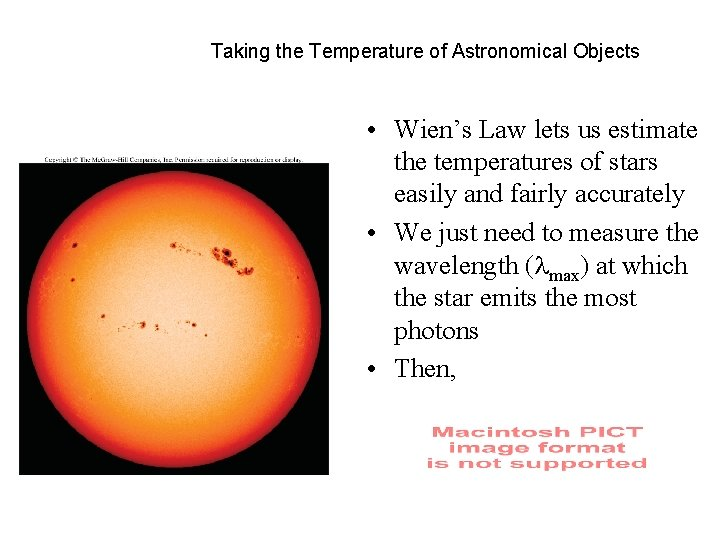Taking the Temperature of Astronomical Objects • Wien’s Law lets us estimate the temperatures of stars easily and fairly accurately • We just need to measure the wavelength ( max) at which the star emits the most photons • Then,The Stefan-Boltzmann Law • If we know an object’s temperature (T), we can calculate how much energy the object is emitting using the SB law • is the Stefan-Boltzmann constant, and is equal to 5. 67 10 -8 Watts/m 2/K 4 • The Sun puts out 64 million watts per square meter – lots of energy!